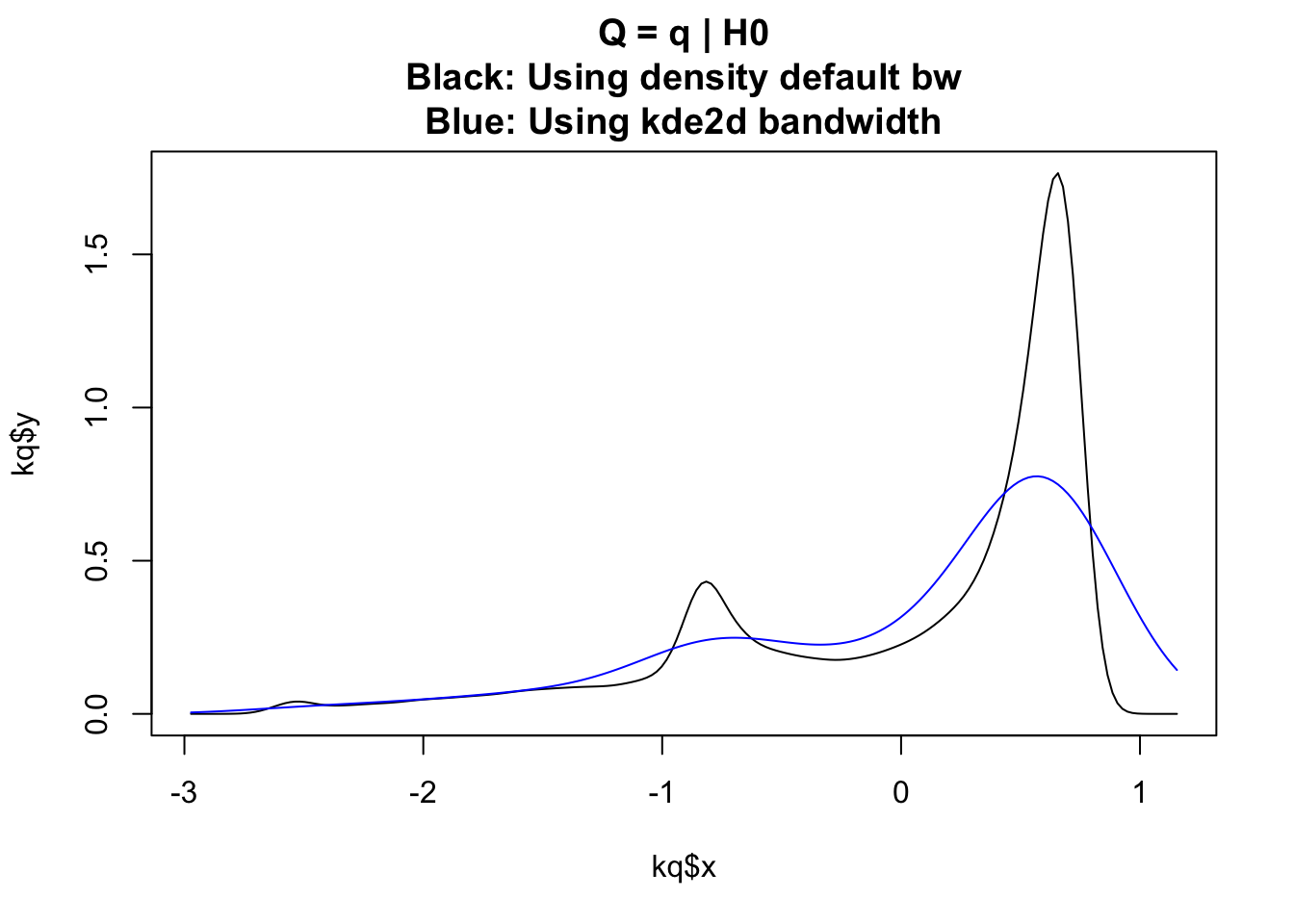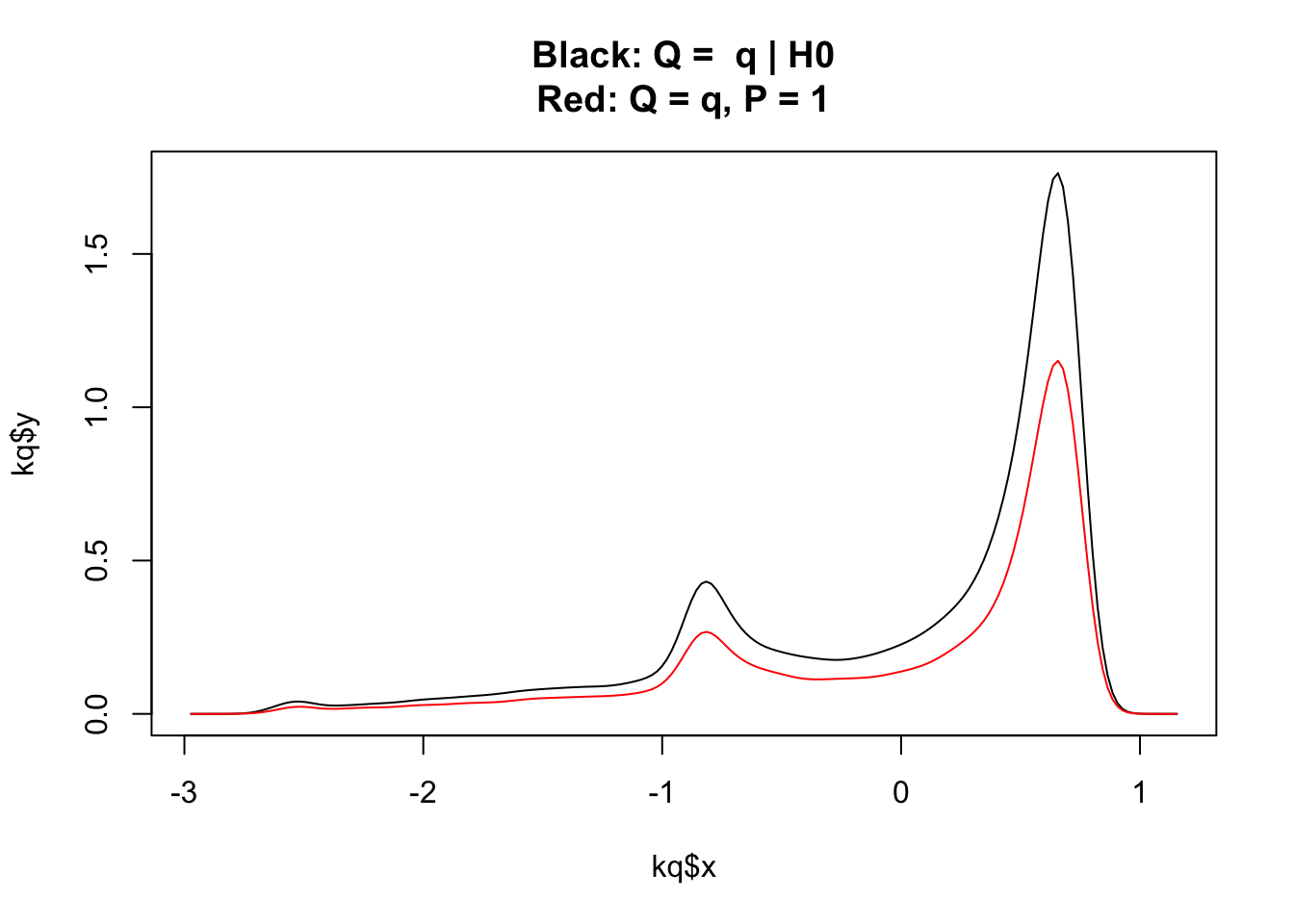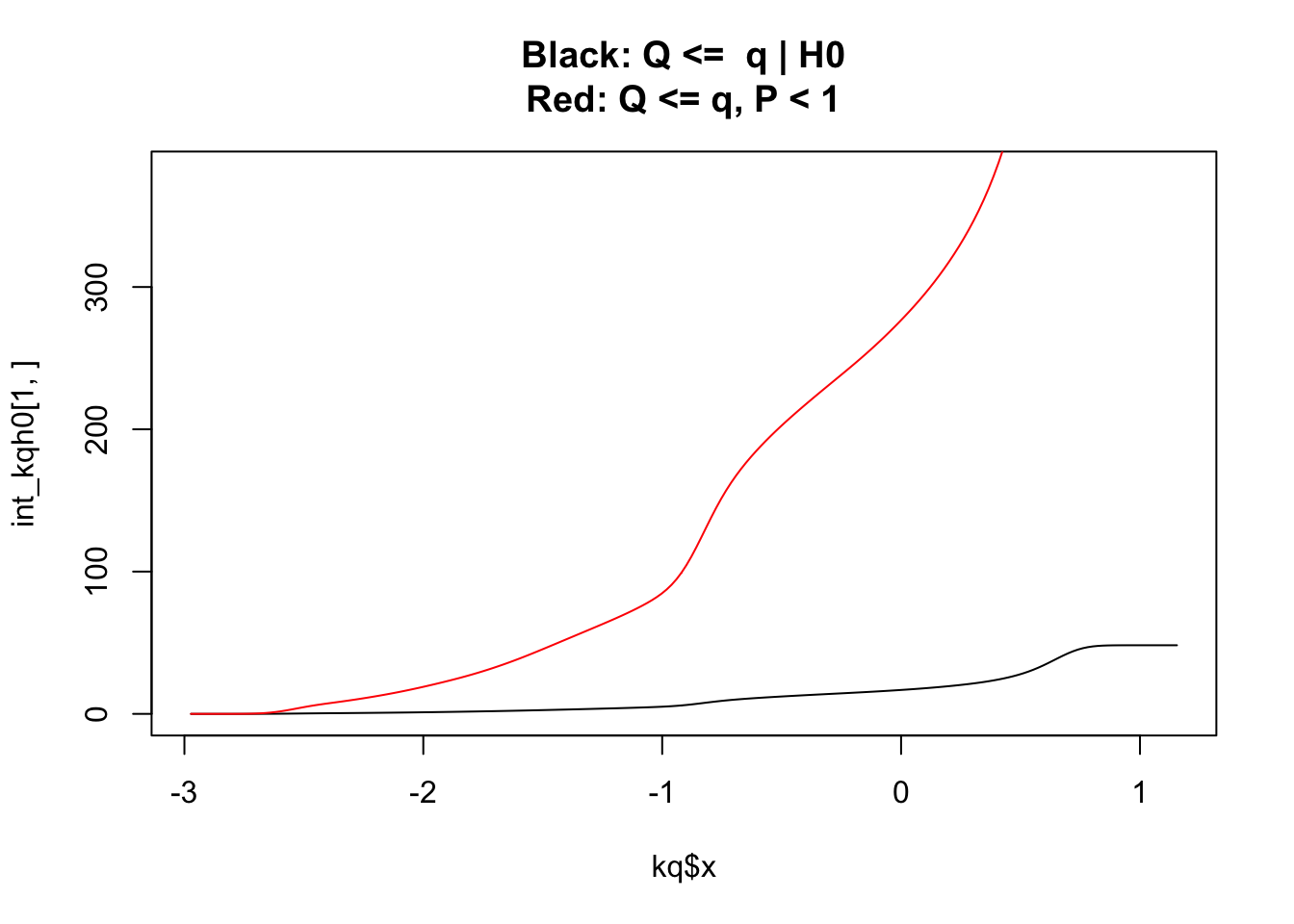### Genomic control

It is important that we use genomic control (or something similar) to ensure that the P values are uniformly distributed under the null so that $$P(P<p)=p$$. If $$P(P<p)>p$$ then we may see inflation of V values. Although note that genomic control is a brute force tool and is not necessarily appropriate for targetted chips, such as the ImmunoChip.

I am unsure whether the P values have already been genomic-controlled. I derive the $$\lambda$$ value using various combinations of SNPs:

lamdbda lambda_1000
Use all SNPs 1.6 1.07
Use null reading SNPs 0.93 0.99
Use SNPs in non-dense regions 1.3 1.03

The only mention of genomic control in the manuscript is (although note here they use 135,870 SNPs that passed QC whereas my dataset only has 124,000 SNPs):

“We observed inflation of test statistics across all SNPs that passed quality control ($$\lambda_{1,000} = 1.09$$), which was expected as the Immunochip was designed to target robustly defined immune-mediated disease susceptibility loci. Excluding SNPs from regions reported here, $$\lambda_{1,000}$$ was reduced to 1.07; excluding all densely genotyped regions reduced $$\lambda_{1,000}$$ to 1.03”

My values are comparable with theirs and I decide not to perform genomic-control on my P values.

### cFDR

I am working on new cFDR code that automatically incorporates the adjusment step.

$\begin{equation} \begin{split} cFDR(p,q) &= P(H_0^p|P\leq p, Q\leq q)\\[2ex] &= \dfrac{P(P\leq p|H_0^p, Q\leq q) P(H_0^p|Q\leq q)}{P(P\leq p |Q\leq q)}\\[2ex] &= \dfrac{P(P\leq p|H_0^p)}{\dfrac{P(P\leq p, Q\leq q)}{P(Q\leq q)}} P(H_0^p|Q\leq q)\\[2ex] &= \dfrac{p P(Q\leq q)}{P(P\leq p, Q\leq q)} \times \dfrac{P(Q\leq q|H_0^p)P(H_0^p)}{P(Q\leq q)}\\[2ex] &\geq \dfrac{p}{P(P\leq p, Q\leq q)} \times P(Q\leq q|H_0^p) \end{split} \end{equation}$

pq_full <- readRDS("realdata.RDS")

p <- pq_full$p q <- pq_full$q

# ensure smaller q are enriched for smaller p
if(cor(p,q,method="spearman") < 0) q <- -q

chr1 = which(pq_full$chr == 1) # problems for very small p values (inflation) # and very big p values (de-inflation) so pick out these candidate_indices = c(which(pq_full$p<0.0005 | pq_full$p>0.99), sample(1:nrow(pq_full), 1000)) indices = intersect(chr1, candidate_indices) fold = chr1 # leave out these indices mode = 2 # leave one-chromosome-out adj = TRUE # new method does adjustment separately - will look at including later nt = 5000 # resolution of L curves (p) nv = 1000 # resolution of L curves (q) res_p = 500 # resolution for KDE estimates res_q = 200 gx = min(p[indices]) zp = -qnorm(p/2) maxx = max(zp) maxy = max(q) miny = min(q) if (mode==2) sub = setdiff(1:length(zp), fold) else sub = 1:length(zp) # define limits of q by adding 10% either side q_10 <- (max(q[sub]) - min(q[sub])) * 0.1 # define upper limit of zp by adding 10% zp_10 <- (max(zp[sub]) - min(zp[sub])) * 0.1 lims <- c(0, maxx + zp_10, miny-q_10, maxy+q_10) # c(xl, xu, yl, yu) # folded normal KDE kpq <- kde2d(c(zp[sub], -zp[sub]), c(q[sub], q[sub]), n=c(res_p, res_q), lims = lims) #h = c(bandwidth.nrd(c(zp[sub], -zp[sub])), bandwidth.nrd(c(q[sub], q[sub])))) qs = q[sub][which(p[sub]>0.5)] kq = density(c(qs, qs), from=lims, to=lims, n=res_q) #bw = bandwidth.nrd(c(qs, qs))) I will look more into this at a later date but note that kde2d and density have different default bandwidths.I ensure that the univariate PDF ($$P(Q=q|H0)$$) integrates to 1: integrate(approxfun(kq$x, kq$y), lims, lims) ## 1.00096 with absolute error < 5.5e-05 kq$y <- kq$y/integrate(approxfun(kq$x, kq$y), lims, lims)$value

I then make the bivariate PDF ($$P(P=p, Q=q)$$) integrate to 1 (I think this is the right thing to do?)

cell_size <- (diff(range(lims, lims)) / res_p) * (diff(range(lims, lims)) / res_q)
integ <- sum(kpq$z) * cell_size integ ##  0.5071539 kpq$z <- kpq$z/integ I compare on a single plot the univariate PDF, $$P(Q=q|H0)$$, and the bivariate PDF for a fixed P at $$P=1$$:I then find the cdfs by summing across rows/columns [Note - should $$P(P\leq1, Q\leq lims)=1$$ and $$P(Q\leq q|H0)=1$$? In which case another standardisation method could be to ensure the top right entry of the cdf matrix is 1]. ##### sum to get P(Q <= q | h0) kqh0 <- t(outer(kq$y, rep(1,res_p)))
int_kqh0 <- t(apply(kqh0, 1, cumsum))

##### sum to get P(P <= p, Q <= q)
int_kpq <- t(apply(apply(kpq$z[res_p:1,], 2, cumsum), 1, cumsum))[res_p:1, ] But now we have an even bigger problem of scale differences….I use a sneaky normalisation trick and define kgrid($$=P(P\leq p, Q\leq q)/P(Q\leq q|H0)$$. int_kqh0 <- int_kqh0 * (max(int_kpq)/max(int_kqh0)) int_kpq[which(int_kpq==0)] = min(int_kpq[which(int_kpq>0)]) # avoid dividing by 0 int_kqh0[which(int_kqh0==0)] = min(int_kqh0[which(int_kqh0>0)]) # avoid dividing by 0 kgrid <- kpq kgrid$z <- int_kpq/int_kqh0